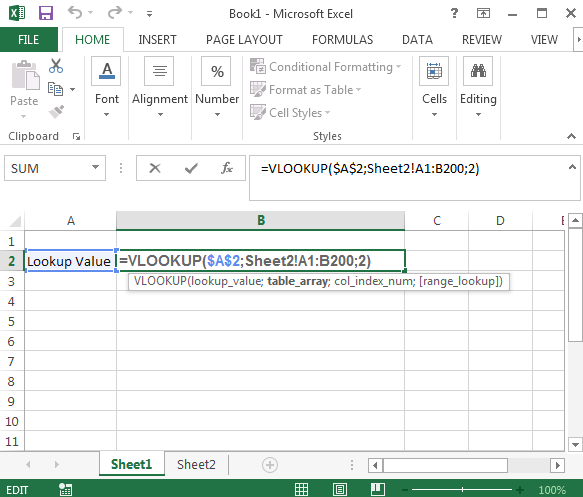#### Vlookup on Multiple Sheets

There are situations when you have data on many sheets. In this lesson you will teach yourself how to do vlookup in this case.

In Excel, you can use the VLOOKUP function to retrieve data from multiple sheets within the same workbook. To do this, you’ll need to specify the sheet name in the `VLOOKUP` formula, followed by an exclamation point (!). Here’s an example of how to perform a VLOOKUP across multiple sheets:

You have your data both in Sheet1 and Sheet2. You want to create a vlookup formula which will analyze data in these two sheets.

## Vlookup many sheets formula

Your formula will look like this:

=VLOOKUP(A2,Sheet2!A1:B200,2)## How it works?

The trick is to write Sheet2!A1:B200 instead of just A1:B200. Sheet2! is the information that you analyze data from the sheet which name is Sheet2.

Lookup value is in A1 cell. You analyze data in Example_Sheet in A1:B10 table array. Number of column is 2. You want to exact match. Your formula is:

=VLOOKUP(A1,Example_Sheet!A1:B10,2,FALSE)

## Vlookup Indirect many sheets formula

Let’s see more advanced example using both Vlookup and Indirect Excel functions.

1. Open your Excel workbook and go to the sheet where you want to retrieve data from multiple sheets.
2. In cell A1, enter the label “Value”.
3. In cell B1, enter the label “Sheet”.
4. In cell C1, enter the label “Result”.
5. In column A, enter the values you want to search for.
6. In column B, enter the names of the sheets where the values are located.
7. In column C, enter the following formula to perform a `VLOOKUP` across multiple sheets: =VLOOKUP(A2,INDIRECT(B2&”!”&”A:B”),2,FALSE)
8. Drag the formula down to the end of the data in column C.
9. The result in column C will be the values from the specified sheets that match the values in column A.
Note: In this example, the `VLOOKUP` function uses the `INDIRECT` function to dynamically reference the sheet name entered in column B. The `VLOOKUP` function then searches for the value in column A in the specified sheet and returns the corresponding value from column B in the same row. The `FALSE` argument in the formula specifies that an exact match is required for the `VLOOKUP` to return a result.